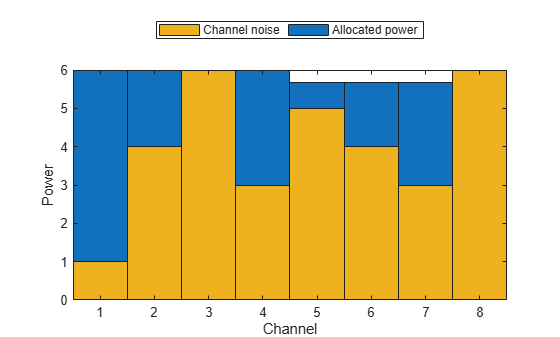# waterfill

Waterfill MIMO power distribution

## Syntax

``P = waterfill(Pt,Pn)``
``waterfill(Pt,Pn)``

## Description

example

````P = waterfill(Pt,Pn)` optimally distributes the total transmitted power, `Pt`, among multiple channels to maximize channel capacity. The argument `Pn` represents the noise in each channel. The function can optimize independent subcarriers simultaneously.```

example

````waterfill(Pt,Pn)` displays a waterfill diagram.```

## Examples

collapse all

Using the waterfill algorithm, compute the distributed power per channel for two subcarriers. There are four channels per subcarrier.

Specify the same total power for both subcarriers using a scalar value.

`Pt = 10;`

Specify the noise power. The rows correspond to the subcarriers and the columns to the channels.

```Pn = [1 4 6 3; 5 4 3 6]; P = waterfill(Pt,Pn)```
```P = 2×4 5 2 0 3 2 3 4 1 ```

Now, specify a different total power for each subcarrier.

```Pt = [10,5]; P = waterfill(Pt,Pn)```
```P = 2×4 5.0000 2.0000 0 3.0000 0.6667 1.6667 2.6667 0 ```

Using the waterfill algorithm, plot the distributed power per channel for two subcarriers. There are four channels per subcarrier.

Specify a different total power for each subcarrier.

`Pt = [10,5];`

Specify the noise power. The rows correspond to the subcarriers and the columns to the channels.

`Pn = [1 4 6 3; 5 4 3 6];`

Display the waterfill plot.

`waterfill(Pt,Pn)`## Input Arguments

collapse all

Total transmitted power per subcarrier, specified as a positive-valued L-element row or column vector where L is the number of subcarriers. When `Pt` is a scalar, all subcarriers have the same power. When `Pt` is a vector, the total power in a subcarriers is given by the corresponding element in `Pt`. Units are arbitrary.

Example: `[20 30]`

Data Types: `double`

Channel noise powers, specified as a positive-valued N-element row or column vector or a positive-valued L-by-N-element matrix. N is the number of channels and L is the number of subcarriers. If `Pn` is a vector, each element represents the noise power in the corresponding channel. The noise powers for each channel is the same for all subcarriers. If `Pn` is a matrix, an element in the matrix represents the noise power in the corresponding channel at the corresponding subcarrier. Units are arbitrary but must match the units for `Pt`.

Example: `[10 20 15]`

Data Types: `double`

## Output Arguments

collapse all

Allocated power per channel, specified as a positive-valued L-by-N-element matrix. N is the number of channels and L is the number of subcarriers. Units are the same as the transmitted power, `Pt`. Each row corresponds to a subcarrier and specifies the distributed power for the channels in the subcarrier. Units are the same as for `Pt` and `Pn`.

Data Types: `double`

## Algorithms

The number of subcarriers is determined by either the dimensions of `Pt` or `Pn`.

• When you specify `Pt` as an L-element vector, there are L subcarriers with different total powers. If you specify `Pn` as N-element vector, this noise power vector is the same for all subcarriers. If you specify `Pn` as an L-by-N matrix, each row applies to the corresponding subcarrier.

• When you specify `Pt` as a scalar, `Pn` determines the number of subcarriers. If you specify `Pn` as an N-element vector, each element is the noise power in a channel and there is only one subcarrier. If you specify `Pn` as an L-by-N matrix, there are L subcarriers all having the same transmitted power.

 Heath, R. Jr. et al. “An Overview of Signal Processing Techniques for Millimeter Wave MIMO Systems”, arXiv.org:1512.03007 [cs.IT], 2015.

 Tse, D. and P. Viswanath, Fundamentals of Wireless Communications, Cambridge: Cambridge University Press, 2005.

 Paulraj, A. Introduction to Space-Time Wireless Communications, Cambridge: Cambridge University Press, 2003.